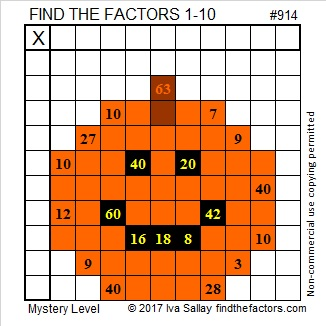# 914 Jack-O’Lantern Mystery Level

25² + 17² = 914, so 914 is the hypotenuse of a Pythagorean triple:
336-850-914, which is 2 times (168-425-457).
You can also calculate it from 2(25)(17), 25² – 17², 25² + 17².

914 becomes 194 in BASE 26. Notice that both bases use the same digits.

914 is also palindrome 494 in BASE 14.

Today’s puzzle is a jack o’lantern that will kick off two weeks worth of Halloween themed puzzles.Print the puzzles or type the solution on this excel file: 10-factors-914-922

• 914 is a composite number.
• Prime factorization: 914 = 2 × 457
• The exponents in the prime factorization are 1 and 1. Adding one to each and multiplying we get (1 + 1)(1 + 1) = 2 × 2 = 4. Therefore 914 has exactly 4 factors.
• Factors of 914: 1, 2, 457, 914
• Factor pairs: 914 = 1 × 914 or 2 × 457
• 914 has no square factors that allow its square root to be simplified. √914 ≈ 30.2324